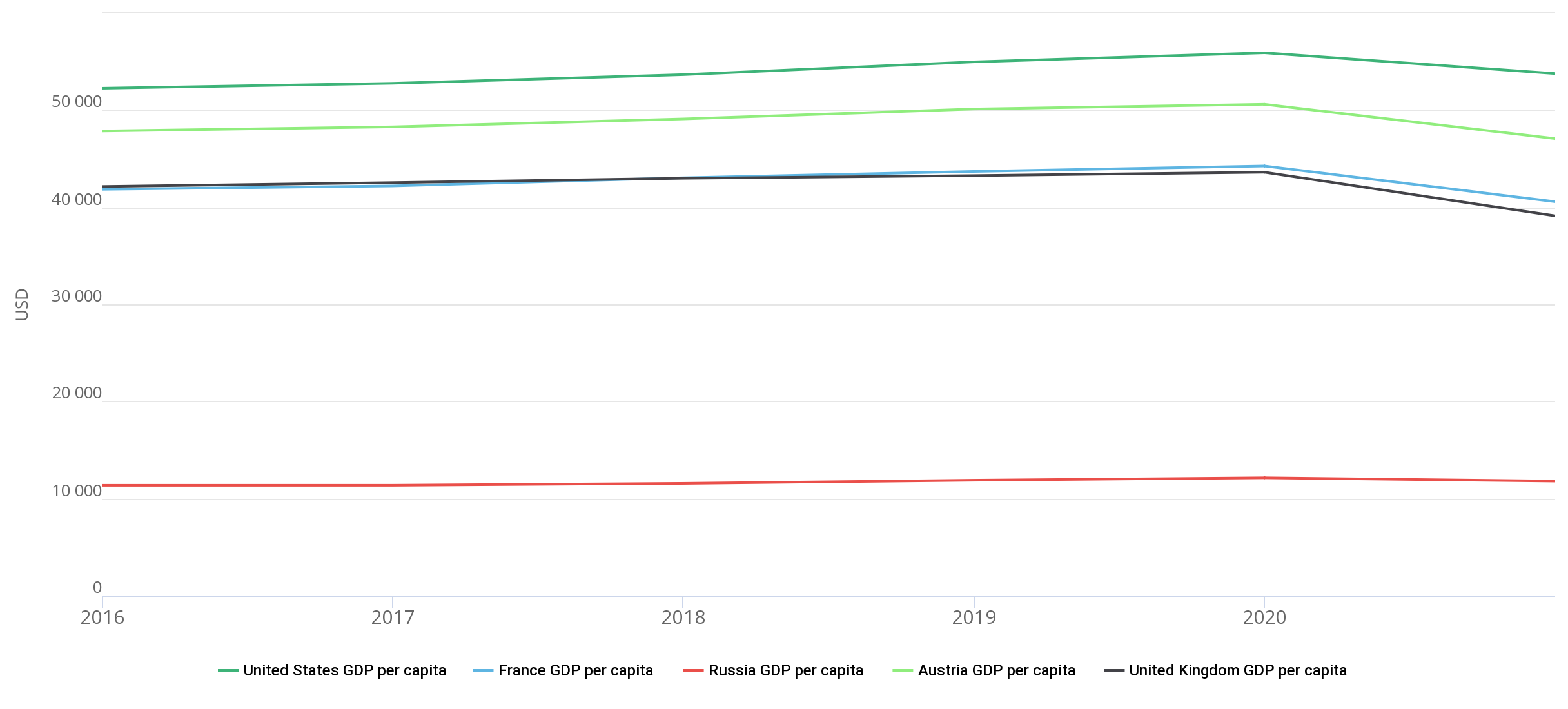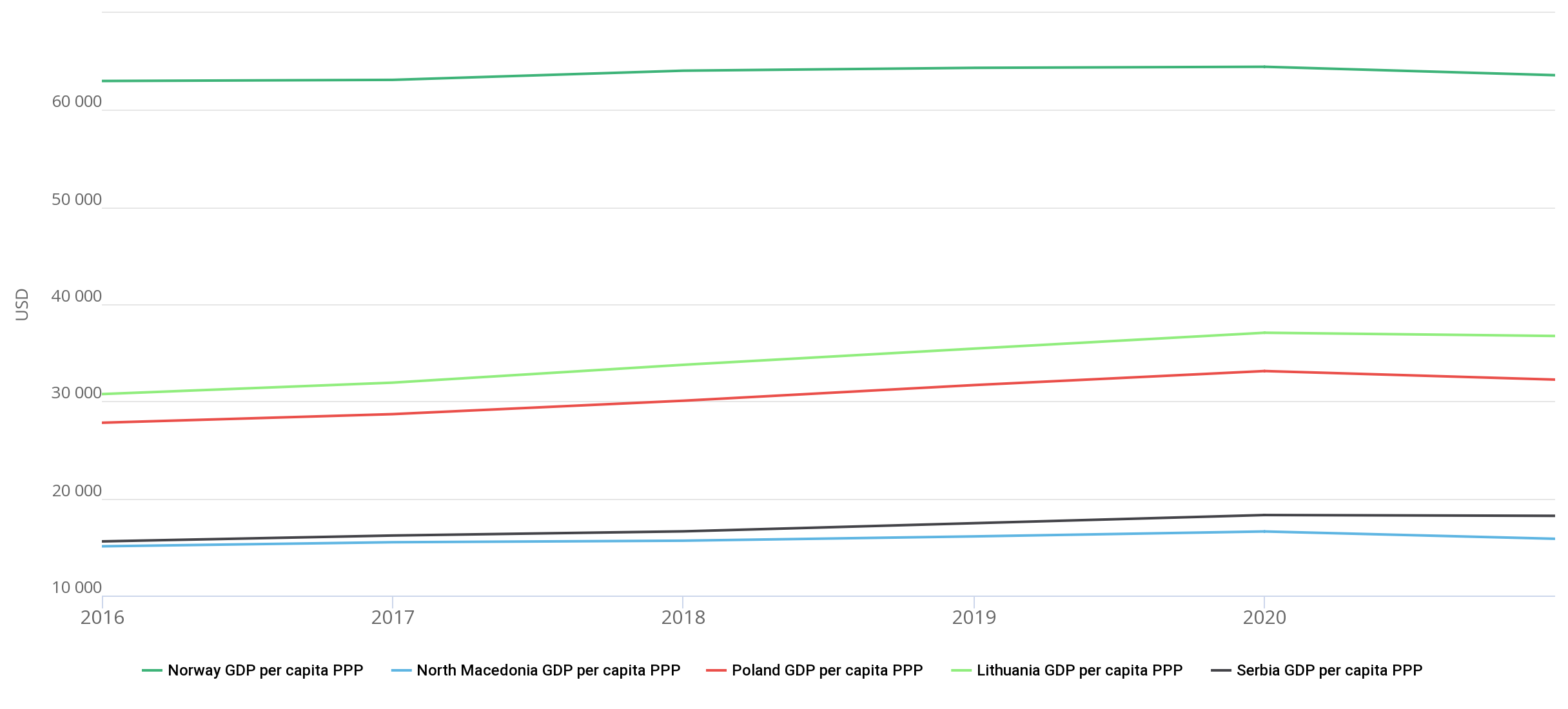Modalità suggerimenti è attiva
Glossario

# GDP per capita | PIL pro capite

GDP per capita is a macroeconomic indicator that is defined as the ratio of a country’s gross domestic product (GDP) to its average annual population.

This indicator is used to assess the level of material well-being of citizens of a certain state and their economic activity for a specific period of time.

GDP per capita is an absolute measure, the values of which are calculated and published on an annual basis.

GDP per capita can be calculated in local currency or world currency (in US dollars). The recalculation of GDP per capita from national currencies into US dollars is made for the convenience of comparing the values of this indicator in different countries.

There are also indicators of real GDP per capita, which is calculated in constant US dollars (for example, GDP per capita in the USA, France, Russia, Austria, and the United Kingdom). The graph shows the dynamics of the values of real GDP per capita in a number of countries. It should be noted that in these countries, the crisis of 2020 led to a decrease in GDP per capita.In addition, in practice, an indicator such as GDP per capita at purchasing power parity (PPP) is often used, which is calculated in international dollars based on purchasing power parity. The latter means the ratio of two currencies, which is established based on prices for the same set of goods in two different countries. At the same time, the international dollar has the same purchasing power as the US dollar.

Examples include GDP per capita based on PPP in North Macedonia, Poland, Lithuania, Serbia, and Norway.

The graph below shows the dynamics of GDP per capita in PPP in the above countries for 2016-2020.Registration is required to get access.